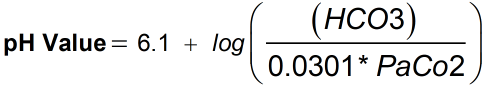Request a Tool

Henderson Calculator

Henderson equation is an approximate equation that shows the relationship between the pH or pOH

pH Value
0

FormulaDefination / Uses

The Henderson equation is an approximation of the relationship between a solution's pH or pOH, the pKa or pKb, and the ratio of dissociated chemical species concentrations. Virginia Henderson, FRCN, was a nurse, researcher, thinker, and author who did live from November 30, 1897 to March 19, 1996. Using the Henderson equation, estimate the isoelectric points of proteins with this calculator.

The pH value of a solution can be calculated using our Henderson calculator. The Henderson-Hasselbalch equation is well-known among clinicians who deal with acid-base imbalances. Unfortunately, most doctors are unaware of the equation's limitations. it has several flaws and is more descriptive than mechanistic. The Henderson-Hasselbalch equation was created separately by L. J. Henderson, an American biological chemist, and K. A. Hasselbalch, a Swedish physiologist, to relate pH to the blood's bicarbonate buffer system. The Henderson equation is a useful statement for buffer calculations in its general form.